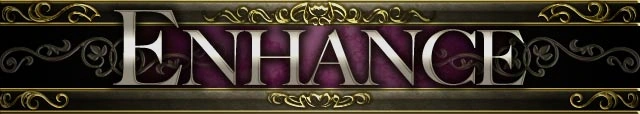## FANDOM

16,852 PagesIn April, 2013, I decided to tackle the problem of the wiki not having a single, comprehensive list of enhancing percentages when skilling. None of the formulae given take all variables into account and thus do not work for any single-card skill enhance of any card. So I began to collect data in order to reverse-engineer a single, all-encompassing formula which can be used to calculate the percent chance shown.

### Introduction

Since it was obvious multiple card enhances showed a percent that was simply the sum of each individual card used, only single-card skilling enhance percentages were necessary to calculate. So I started collecting data to fill in a table and asked guild members to feed me a few high skill level data points I did not have the cards to obtain myself. As time went on, data accumulated and patterns emerged. A brute force attack on the problem taking all variables into account at once seemed to me the easiest way to accomplish the task, although looking back, I probably could have saved time if I piece-wised the problem.

A month later, came the “eureka!” day in which, from the few known, scattered data points, a script was written that calculated all known values correctly. However, anyone wanting to calculate a value would have to be comfortable with a UNIX terminal, making my script unwieldy at its best, incomprehensible at its worst. I found using this formula was much more of a hinderance than a benefit. To get around this, I used my formula to calculate every single-card skill enhance possibility and filled in the the data points, resulting in the skilling tables below.

For about three months, I never saw (nor did my guild report) a discrepancy between calculated values and game values. After such a time, I presume the values are correct.

### Definitions

The tables use a common language. The two most important concepts are:

• Enhancee: the card you are attempting to skill up
• Enhancer: the card burned in the skilling process

### Color Coding

The two words are almost identical and each has certain characteristics that are variables in the formula. To further complicate matters, these characteristics are usually identically named (eg. Enhancee rarity/Enhancer rarity). Thus, in an attempt to make the tables easier to read, everything is color-coded as follows:

• White text on dark background: enhancee characteristics
• Yellow text on red background: enhancer characteristics

### The Formula

Thanks goes out to Cancan for the generation of this single, all encompassing formula for calculating the skill up percent. The original is in the comments section, but I've syntax-colored it, and made a few other modifications so it can be easier visualized:

`[10/(t×(max(1,2(e−4))))]×(s+1)×[z+min(1,5−e)×(max(0,5−e))/(max(1,5−e))×max(4−e,z−2×(e−1))`[more]
[continued]`+max(0,e−4)×max(0,6−e)×max(0,z−2)+max(0,e−5)×max(0,1+3(z−3))]`

Take my word for it, this one is a lot simpler than the one I used.

I have taken the further liberty to completely re-arrange the above formula again into a form more easily readable at a glance. I also moved the constant `10` into the denominator so the formula results in a probability value. Here it is, courtesy of Wolfram Mathematica for OSX:

The reason for its complexity? There are simply too many variables which need to be taken into account. This is a 4-dimensional formula translated into a 1-dimensional representation.

### Variables

For the tables to show every possibility of single-card skill enhances, they must show every possibility of every variable characteristic. The variables which affect the percent chance when skilling are as follows:

• eEnhancee rarity: 6 possibilities
• tEnhancee current/target skill level: 9 possibilities
• zEnhancer rarity: 6 possibilities
• sEnhancer skill level: 10 possibilities

Since each of the variables is not dependent on any of the other variables, a simple multiplication (`6×9×6×10`) reveals `3240` possible single-card enhance pairs, each having a different percent chance to bump the skill level up. As a result, the calculated percents have necessarily been split up into many tables; a single, all-encompassing table would be far too difficult to read.

### Table Formats

A table is simply a heirarchically-formatted visual representation of all values in a set of data. Every variable accounted for adds (or is) a level in the hierarchy in the same way each variable adds (or is) a dimension in a graphical representation. Given 4 variables, there is `24 = 16` possible ways to format the data into tables (actually, because each variable is independent, x and y can be assigned somewhat arbitrarily, adding another level: `25 = 32` possible formats). Because of this, the tables can be presented in a variety of formats for all variables to be shown.

In order to minimize the number of tables, the top-level in the heirarchy should have the fewest possibilities, making it a choice between enhancee rarity or enhancer rarity. I have chosen the former because it is more constant, so less table shuffling. That leaves the other three levels to be placed wherever.

The two layouts most beneficial for comparisons I have converted into html/css with the colors of this wiki. Click on the links below or below to view the tables:Enhancee rarity ↳ Enhancer skill level ↳ Enhancee current skill level ↳ Enhancer rarity
 Enhancee rarity ↳ Enhancee current skill level ↳ Enhancer rarity ↳ Enhancer skill level
 • Ultra Rare EX • Ultra Rare • Rare EX • Rare • Common EX • Common
 • Ultra Rare EX • Ultra Rare • Rare EX • Rare • Common EX • Common